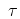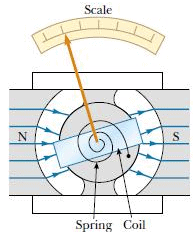Courses

# Notes | EduRev

## JEE Main Mock Test Series 2020 & Previous Year Papers

Created by: Learners Habitat

## JEE : Notes | EduRev

The document Notes | EduRev is a part of the JEE Course JEE Main Mock Test Series 2020 & Previous Year Papers.
All you need of JEE at this link: JEE

Question 1: Suppose a cyclotron is operated at an oscillator frequency of 12 MHz and has a dee radius R = 53 cm.
(a) What is the magnitude of the magnetic field needed for deuterons to be accelerated in the cyclotron?
(b) What is the resulting kinetic energy of the deuterons?
Solution:-
(a) A deuteron has the same charge as a proton but approximately twice the mass (m = 3.34×10-27 kg). From equation qB = 2πmfosc,
B = 2πmfosc/q
= [(2π) (3.34×10-27kg)(12×106s-1)]/[1.6×10-19C]
= 1.57 T
Note that, to allow protons to be accelerated, B would have to be reduced by a factor of 2, assuming that the oscillator frequency remained fixed at 12 MHz.
(b) From equation r = mv/qB, the speed of the deuteron circulating with a radius equal to the dee radius R is given by
v = RqB/m
= [(0.53 m)(1.6×10-19C)(1.57 T)]/[3.34×10-27kg]
=3.99×107 m/s
This speed corresponds to a kinetic energy of
K = ½ mv2
= ½ (3.34×10-27kg)(3.99×107 m/s)2×(1MeV/1.60×10-13J)
= 16.6 MeV
From the above observation we conclude that, the resulting kinetic energy of the deuterons would be 16.6 MeV.

Question 2: Analog voltmeters and ammeters work by measuring the torque exerted by a magnetic field on a current carrying coil. The reading is displayed by means of the deflection of a pointer over a scale. Below figure shows the basic galvanometer, on which both analog ammeters and analog voltmeters are based. The coil is 2.1 cm high and 1.2 cm wide; it has 250 turns and is mounted so that it can rotate about an axis (into the page) in a uniform radial magnetic field with B = 0.23 T. For any orientation of the coil, the net magnetic field through the coil is perpendicular to the normal vector of the coil. A spring provides a counter torque that balances the magnetic torque, so that a given steady current i in the coil results in a steady angular deflection ?. If a current of 100 µA produces an angular deflection of 28°, what must be the torsional constant κ of the spring, as used in equation= -κ??Solution:
Setting the magnetic torque [Equation,= (NiA) B sinθ] equal to the spring torque and using absolute magnitudes yield= NiA B sinθ = κ?,
in which ? is the angular deflection of the coil and pointer, and A (=2.52×10-4 m2) is the area encircled by the coil. Since the net magnetic field through the coil is always perpendicular to the normal vector of the coil, θ = 90° for any orientation of the pointer.
Solving the equation= NiA B sinθ = κ? for κ, we find
κ = NiA B sinθ/?
= (250)(100×10-6 A) (2.52×10-4 m2)× [(0.23 T) (sin 90°)/28°]
= 5.2×10-8 N.m/degree
Many modern ammeters and voltmeters are of the digital, direct-reading type and operate in a way that does not involve a moving coil.

Question 3: (a) What is the magnetic dipole moment of the coil of Question 2, assuming that it carries a current of 100 µA?
(b) The magnetic dipole moment of the galvanometer coil is lined up with an external magnetic field whose strength is 0.85 T. How much work would be required to turn the coil end for end?
(c) What is the magnitude of the maximum torquethat the external field B can exert on the magnetic dipole moment?
Solution:-
(a) The magnitude of the magnetic dipole moment of the coil, whose area A is 2.52×10-4 m2, is
μ = NiA
= (250) (100×10-6A) (2.52×10-4 m2)
= 6.3×10-6 A. m2
= 6.3×10-6 J/T
Therefore, the magnetic dipole moment of the coil would be 6.3×10-6 J/T. You can show that these two sets of units are identical.
(b) The required work is equal to the increase in potential energy; that is, from equation ΔU = 2µB,
W = ΔU
= 2µB
= 2(6.3×10-6 J/T) (0.85 T)
= 10.7×10-6 J
≈ 11 µJ
From the above observation we conclude that, the work required to turn the coil end for end would be11 µJ.
(c) From equation=μB sinθ, the maximum torque occurs when the magnitude of sinθ is 1. Thus, we have=μB sinθ
= (6.3×10-6 J/T) (0.85 T)(1)
=  5.4×10-6 N.m
Thus the maximum torquethat the external field B can exert on the magnetic dipole moment would be 5.4×10-6 N.m.

Offer running on EduRev: Apply code STAYHOME200 to get INR 200 off on our premium plan EduRev Infinity!

## JEE Main Mock Test Series 2020 & Previous Year Papers

3 videos|174 docs|149 tests

,

,

,

,

,

,

,

,

,

,

,

,

,

,

,

,

,

,

,

,

,

;